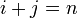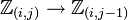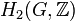# Group cohomology of Klein four-group

View group cohomology of particular groups | View other specific information about Klein four-group

## Classifying space and corresponding chain complex

The classifying space of the Klein four-group is the product space$\mathbb{R}\mathbb{P}^\infty \times \mathbb{R}\mathbb{P}^\infty$, where$\mathbb{R}\mathbb{P}^\infty$ is infinite-dimensional real projective space.

A chain complex that can be used to compute the homology of this space is given as follows:

• The$n^{th}$ chain group is a sum of$n + 1$ copies of$\mathbb{Z}$, indexed by ordered pairs$(i,j)$ where$i + j = n$. In other words, the$n^{th}$ chain group is:$\mathbb{Z}_{(0,n)} \oplus \mathbb{Z}_{(1,n-1)} \oplus \dots \mathbb{Z}_{(n,0)}$

• The boundary map is (up to some sign issues that need to be fixed!) given by adding up the following maps:
• The map$\mathbb{Z}_{(i,j)} \to \mathbb{Z}_{(i,j-1)}$ is multiplication by zero if$j$ is odd and is multiplication by two if$j$ is even.
• The map$\mathbb{Z}_{(i,j)} \to \mathbb{Z}_{(i-1,j)}$ is multiplication by zero if$i$ is odd and multiplication by two if$i$ is even.

## Homology groups

### Over the integers

The homology groups with coefficients in the ring of integers$\mathbb{Z}$ are given as follows:$H_p(\mathbb{Z}/2\mathbb{Z} \oplus \mathbb{Z}/2\mathbb{Z};\mathbb{Z}) = \left\lbrace\begin{array}{rl} (\mathbb{Z}/2\mathbb{Z})^{(p + 3)/2}, & p = 1,3,5,\dots \\ (\mathbb{Z}/2\mathbb{Z})^{p/2}, & p = 2,4,6,\dots \\ \mathbb{Z}, & p = 0 \\\end{array}\right.$

These homology groups can be obtained from the knowledge of the homology groups of cyclic group:Z2 (see group cohomology of cyclic group:Z2) using the Kunneth formula for group homology. They can also be computed explicitly using the chain complex description above.

Here is the computation using the Kunneth formula for group homology: <toggledisplay>

We set$G_1 = G_2 = \mathbb{Z}/2\mathbb{Z}$ and$M = \mathbb{Z}$ in the formula.

Case on$p$ Value of$H_i(G_1;M) \otimes H_j(G_2;M)$ where$i + j = p$ Value of$\operatorname{Tor}_{\mathbb{Z}}^1(H_u(G_1;M),H_v(G_2;M))$ where$u + v = p -1$ Value of$\bigoplus_{i+j = p} H_i(G_1;M) \otimes H_j(G_2;M)$ Value of$\bigoplus_{u + v = p - 1} \operatorname{Tor}_{\mathbb{Z}}^1(H_u(G_1;M),H_v(G_2;M))$ Value of$H_p(G_1 \times G_2;M)$ (sum of preceding two columns$p = 0$$\mathbb{Z}$ in case$i = j = 0$ No such cases$\mathbb{Z}$ 0$\mathbb{Z}$$p$ odd positive$\mathbb{Z}/2\mathbb{Z}$ for the case$i = 0, j = p$ and the case$i = p, j = 0$. 0 in all other cases, because for$i + j$ to be odd, at least one of$i$ and$j$ must be even, forcing the corresponding homology group to be 0.$\mathbb{Z}/2\mathbb{Z}$ when$u,v$ are both odd positive, 0 otherwise$\mathbb{Z}/2\mathbb{Z} \oplus \mathbb{Z}/2\mathbb{Z}$$(\mathbb{Z}/2\mathbb{Z})^{(p-1)/2}$ because that's the number of ordered pairs of positive odd numbers that add up to$p - 1$.$(\mathbb{Z}/2\mathbb{Z})^{(p+3)/2}$$p$ even positive$\mathbb{Z}/2\mathbb{Z}$ for the cases$i,j$ both odd positive, 0 otherwise. zero in all cases$(\mathbb{Z}/2\mathbb{Z})^{p/2}$ 0$(\mathbb{Z}/2\mathbb{Z})^{p/2}$

### Over an abelian group$M$

The homology groups with coefficients in an abelian group$M$ (which may be equipped with additional structure as a module over a ring$R$) are given as follows:

## Cohomology groups and cohomology ring

### Groups over the integers

The cohomology groups with coefficients in the integers are given as below:$H^p(\mathbb{Z}/2\mathbb{Z} \oplus \mathbb{Z}/2\mathbb{Z};\mathbb{Z}) = \left\lbrace \begin{array}{rl} (\mathbb{Z}/2\mathbb{Z})^{(p+1)/2}, & p = 1,3,5,\dots \\ (\mathbb{Z}/2\mathbb{Z})^{(p+2)/2}, & p = 2,4,6,\dots \\ \mathbb{Z}, & p = 0 \\\end{array}\right.$

## Second cohomology groups and extensions

### Schur multiplier

The Schur multiplier, defined as the second cohomology group for trivial group action$H^2(G,\mathbb{C}^\ast)$ and also as the second homology group$H_2(G,\mathbb{Z})$, is isomorphic to cyclic group:Z2.